Function Repository Resource:

# TranslationSurface

Compute a translation surface parametrization

Contributed by: Wolfram Staff (original content by Alfred Gray)
 ResourceFunction["TranslationSurface"][c1,c2,{t,u,v}] computes a translation surface parametrized by u and v from two curves c1 and c2 parametrized by t.

## Details and Options

A translation surface is the surface formed by moving the curve c1 (first generatrix) parallel to itself such that a point of the curve moves along the curve c2 (second generatrix), giving the surface formed by the parallel translation.
ResourceFunction["TranslationSurface"] computes a translation surface from curves c1 and c2 depending on t with a parametrization in terms of variables u and v.

## Examples

### Basic Examples (7)

A plane is the translation surface of two intersecting lines:

 In:=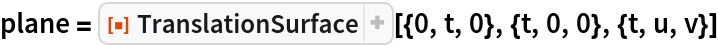Out=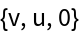Plot it:

 In:=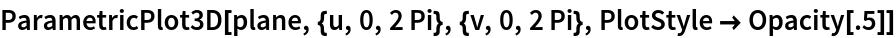Out=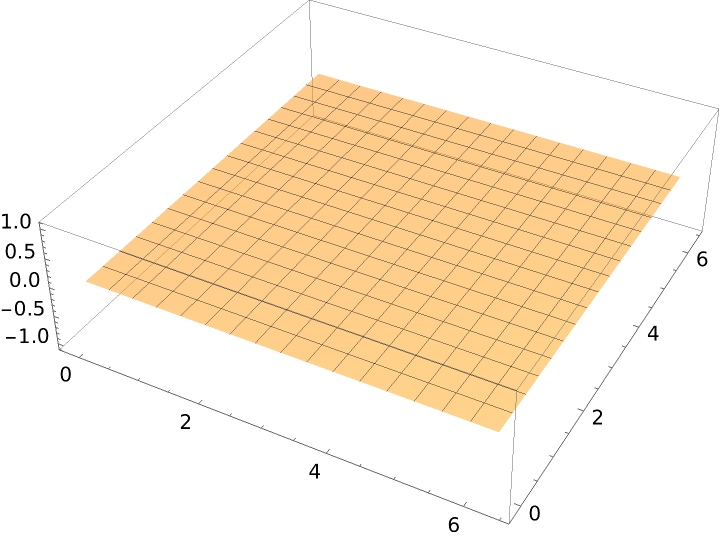A cylinder is the translation surface of a line translated along a circle:

 In:=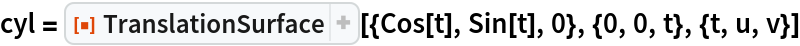Out=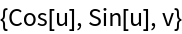Plot it:

 In:=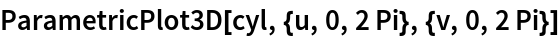Out=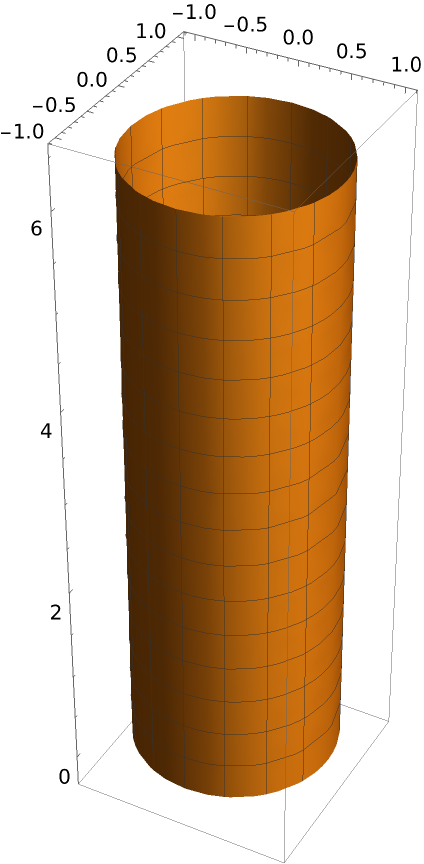A circle translated along a cosine curve:

 In:=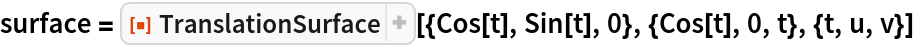Out=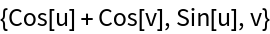Plot it:

 In:=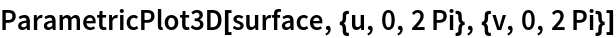Out=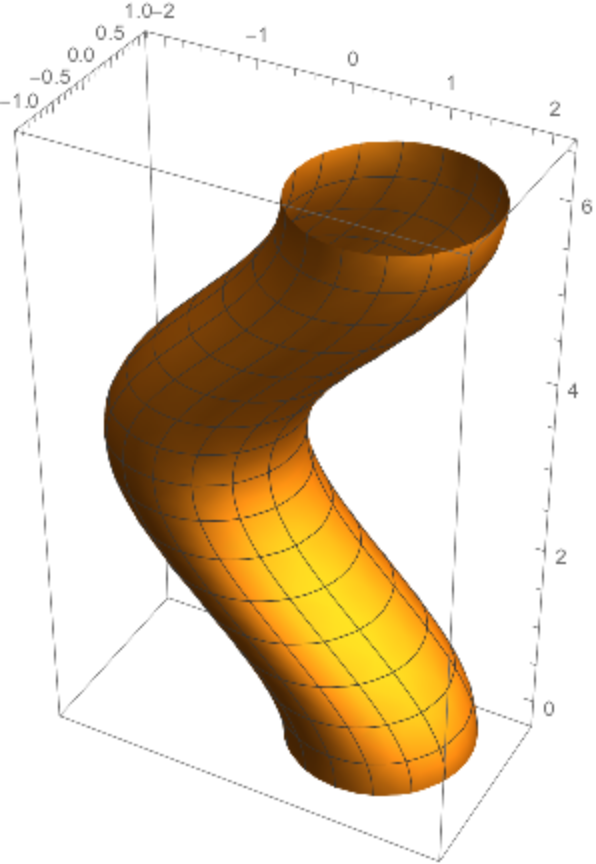Form elliptic and hyperbolic paraboloids by translating one parabola along another:

 In:=Out=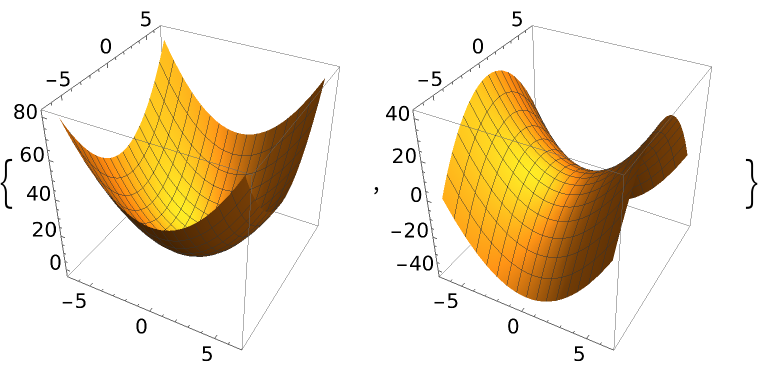Translate one helix (red) along another helix (blue):

 In:=Out=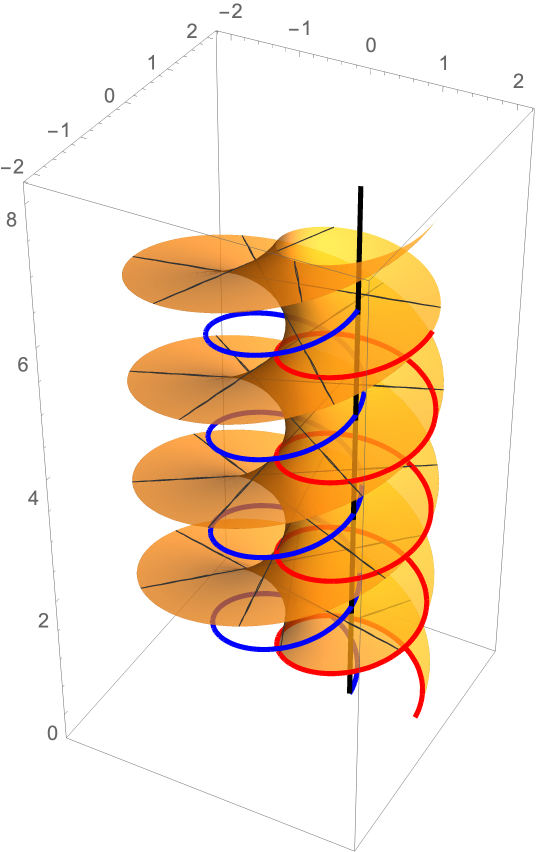Form an egg box surface by translating one sine along another:

 In:=Out=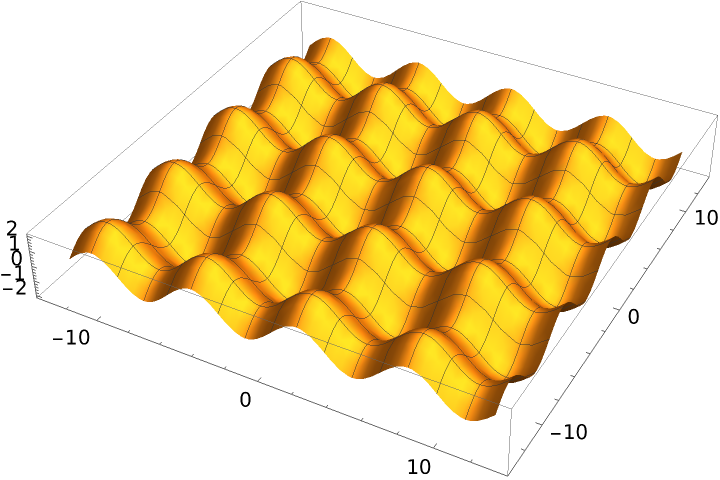For the Bohemian dome, the two generatrices are circles:

 In:=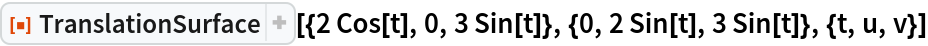Out=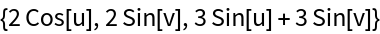In:=Out=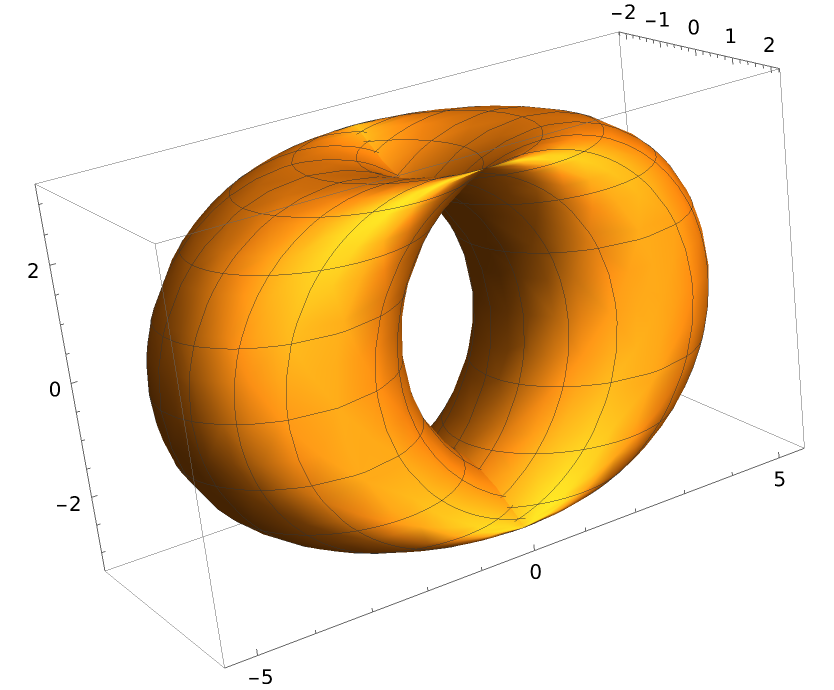### Properties and Relations (5)

A sample surface:

 In:=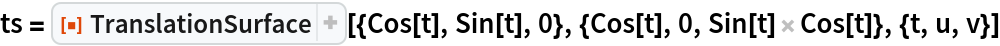Out=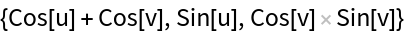For the coefficients of the second fundamental form, the second coefficient f is equal to zero for a translation surface:

 In:=Out=A surface generated by a polynomial translated along a parabola:

 In:=Out=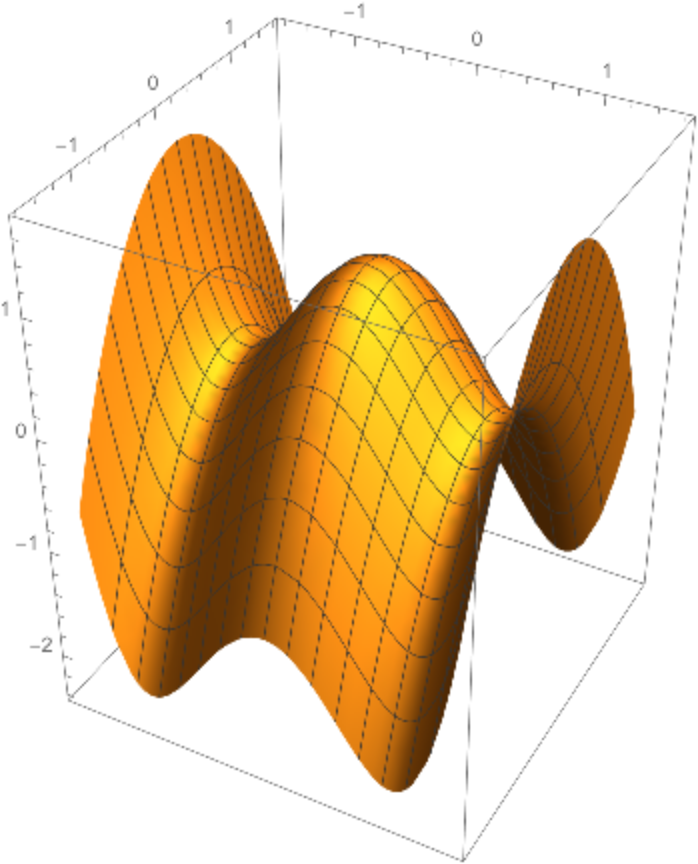There is symmetry as when the order of the curves is reversed, the same surface is obtained:

 In:=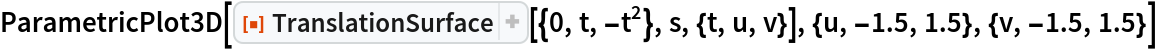Out=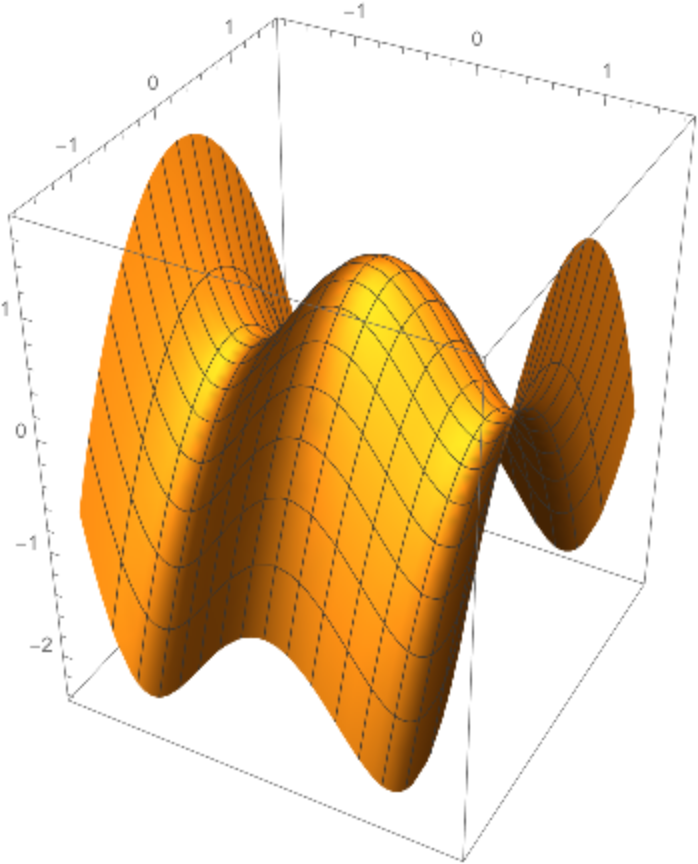This surface is equivalent to the surface of revolution of the sinusoid:

 In:=Out=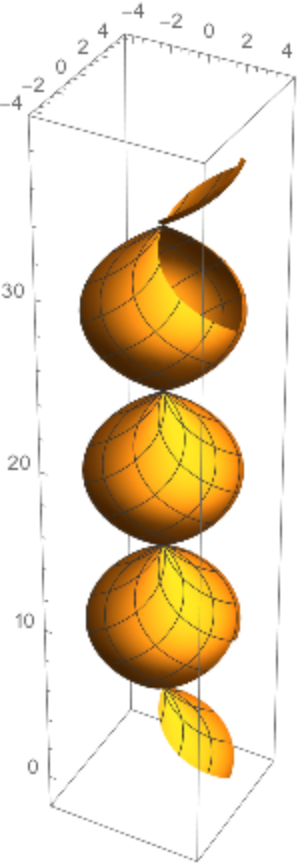The first Scherk minimal surface:

 In:=Out=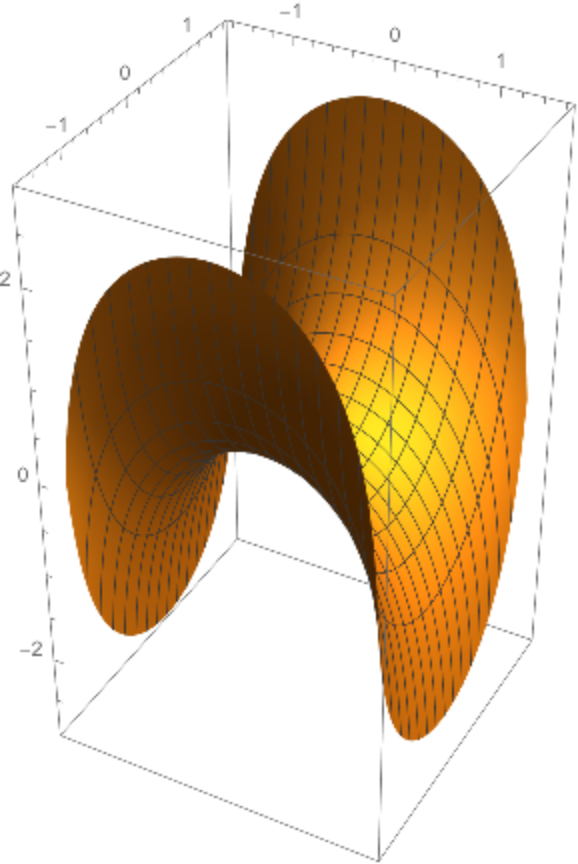Code to get a curve with prescribed curvature (intrinsic curvature):

 In:=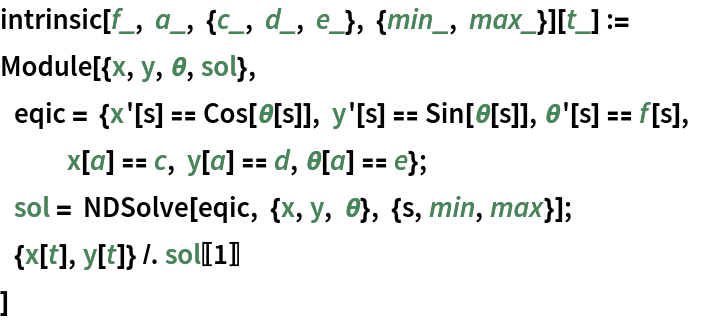Choose two functions:

 In:=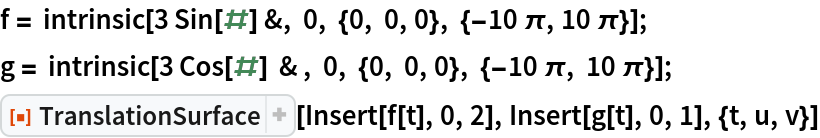Out=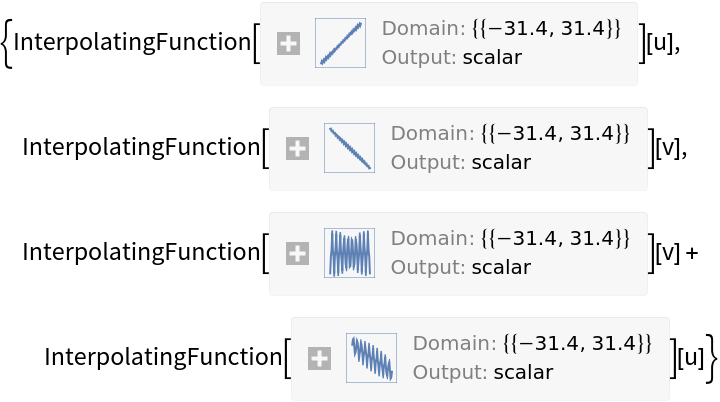Plot the surface:

 In:=Out=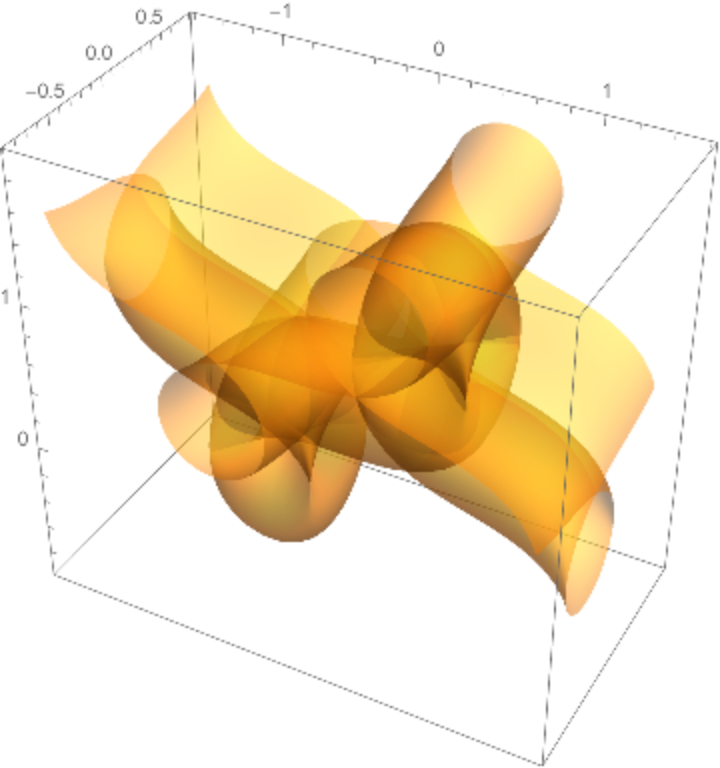Enrique Zeleny

## Version History

• 1.0.0 – 22 June 2020